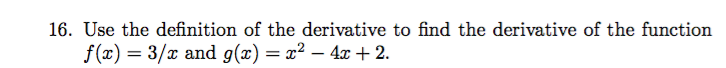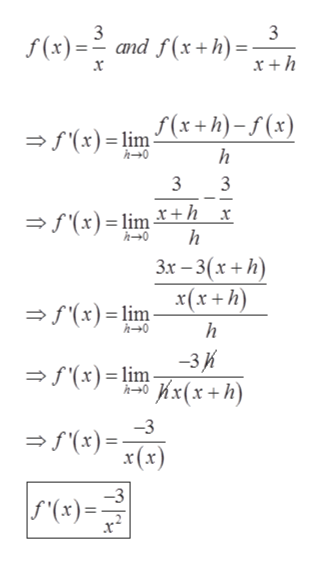# 16. Use the definition of the derivative to find the derivative of the functionf(x) = 3/x and g(x) = x² – 4 + 2.

Question
25 viewshelp_outlineImage Transcriptionclose16. Use the definition of the derivative to find the derivative of the function f(x) = 3/x and g(x) = x² – 4 + 2. fullscreen
check_circle

Step 1

Given,

Step 2

Definition of derivative say that  - The derivative of function f(x) with respect to x is

Step 3

Part (a)...help_outlineImage Transcriptionclose3 S(x) = and f(x+h) = %3D x +h f(x+h)- f (x) = f'(x)= lim 3 = f'(x) = lim r+h__x 3x – 3(x+h) x(x+h) = f'(x) = lim -3K * hx(x+h) =f (x)= lim -3 a f'(x)= x(x) -3 S'(x)=- x* fullscreen

### Want to see the full answer?

See Solution

#### Want to see this answer and more?

Solutions are written by subject experts who are available 24/7. Questions are typically answered within 1 hour.*

See Solution
*Response times may vary by subject and question.
Tagged in

### Calculus